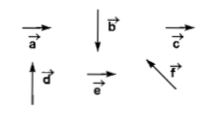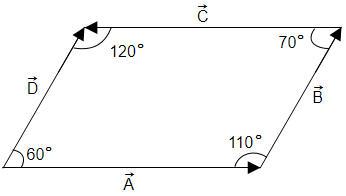You’ve reached the end of your free Videos limit.
#22 | Vectors : Subtraction
(Physics) > Mathematical Tools
Unable to watch the video, please try another server
Related Practice Questions :

Given that  makes an angle . Which of the following options is correct?

1.

2.

3.

4.

High Yielding Test Series + Question Bank - NEET 2020

Difficulty Level:

Two forces are such that the sum of their magnitudes is 18 N and their resultant is perpendicular to the smaller force and magnitude of resultant is 12 N. Then the magnitudes of the forces are

1. 12 N, 6 N

2. 13 N, 5N

3. 10 N, 8 N

4. 16 N, 2 N

High Yielding Test Series + Question Bank - NEET 2020

Difficulty Level:

If the magnitude of sum of two vectors is equal to the magnitude of difference of the two vectors, the angle between these vectors is

1. 90°

2. 45°

3. 180°

4. 0°

High Yielding Test Series + Question Bank - NEET 2020

Difficulty Level:

Six vectors  have the directions indicated in the figure. Which of the following statements may be true?(a) $\stackrel{\to }{b}+\stackrel{\to }{c}=\stackrel{\to }{-f}$                        (b) $\stackrel{\to }{d}+\stackrel{\to }{c}=\stackrel{\to }{f}$

(c) $\stackrel{\to }{d}+\stackrel{\to }{e}=\stackrel{\to }{f}$                        (d) $\stackrel{\to }{b}+\stackrel{\to }{e}=\stackrel{\to }{f}$

High Yielding Test Series + Question Bank - NEET 2020

Difficulty Level:

In the given figure(A)  Angle between $\stackrel{\to }{\mathrm{A}}$ and $\stackrel{\to }{\mathrm{B}}$ is $110°$

(B)  Angle between $\stackrel{\to }{\mathrm{C}}$ and $\stackrel{\to }{\mathrm{D}}$ is $60°$

(C)  Angle between $\stackrel{\to }{\mathrm{B}}$ and $\stackrel{\to }{\mathrm{C}}$ is $110°$

(D)  Angle between $\stackrel{\to }{\mathrm{B}}$ and $\stackrel{\to }{\mathrm{C}}$ is $70°$